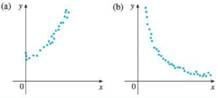Chapter 1.2, Problem 22E### Single Variable Calculus: Early Tr...

8th Edition
James Stewart
ISBN: 9781305270343

#### Solutions

Chapter
Section### Single Variable Calculus: Early Tr...

8th Edition
James Stewart
ISBN: 9781305270343
Textbook Problem

# For each scatter plot, decide what type of function you might choose as a model for the data. Explain your choices.22.(a)

To determine
The type of function which is more appropriate for the given scatter plot and justify the reason of your choice.
Explanation

Reason:

From the given scatter plot, it is observed that the increasing pattern but not in the form of straight line.

Thus, the function is of the type of exponential function...

(b)

To determine
The type of function which is more appropriate for the given scatter plot and justify the reason of your choice.

### Still sussing out bartleby?

Check out a sample textbook solution.

See a sample solution

#### The Solution to Your Study Problems

Bartleby provides explanations to thousands of textbook problems written by our experts, many with advanced degrees!

Get Started